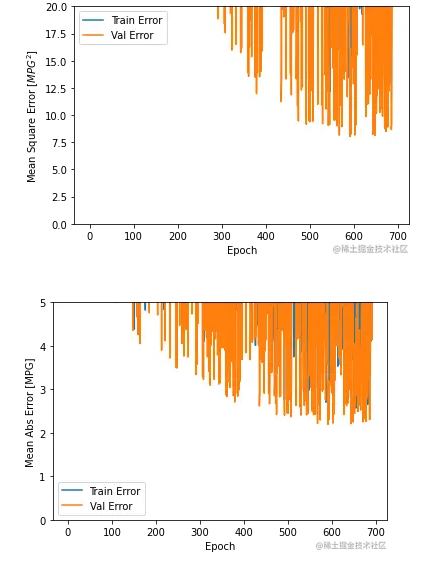﻿ Tensorflow 2.1完成对MPG回归预测详解_python_脚本之家
python# Tensorflow 2.1完成对MPG回归预测详解

## 获取 Auto MPG 数据并进行数据的归一化处理

（1）Auto MPG 数据集描述了汽车燃油效率的特征值和标签值，我们通过模型的学习可以从特征中找到规律，最后以最小的误差来预测目标 MPG 。

（2）我们使用 keras 自带的函数可以直接从网络上下载数据保存到本地。

（3）每行都包含 MPG 、气缸、排量、马力、重量、加速、车型年份、原产地等八列数据，其中 MPG 就是我们的标签值，其他都是特征。

```dataset_path = keras.utils.get_file("auto-mpg.data", "http://archive.ics.uci.edu/ml/machine-learning-databases/auto-mpg/auto-mpg.data")
column_names = ['MPG','气缸','排量','马力','重量', '加速', '车型年份', '原产地']
raw_dataset = pd.read_csv(dataset_path, names=column_names, na_values = "?", comment='\t',  sep=" ", skipinitialspace=True)
dataset = raw_dataset.copy()
```

## 对数据进行处理

（1）因为数据中存在一些空值，会影响我们对于特征的计算和目标的预测，所以将数据中存在空数据的行删除掉。

```dataset = dataset.dropna()
```

（2）因为“原产地”这一列总共只有 1、2、3 三种值，分别表示三个国家，所以我们将他们各自提出来单独做成一列，这样就相当于给每个国家类别转换成了 ont-hot 。

```origin = dataset.pop('原产地')
dataset['阿美莉卡'] = (origin == 1)*1.0
dataset['殴们'] = (origin == 2)*1.0
dataset['小日本子'] = (origin == 3)*1.0
```

（3）按照一定的比例，取 90% 的数据为训练数据，取 10% 的数据为测试数据。

```train_datas = dataset.sample(frac=0.9, random_state=0)
test_datas = dataset.drop(train_dataset.index)
```

（4） 这里主要是使用一些内置的函数来查看训练集对每一列数据的各种常见的统计指标情况，主要有 count、mean、std、min、25%、50%、75%、max ，这样省去了我们后边的计算，直接使用即可。

```train_stats = train_datas.describe()
train_stats.pop("MPG")
train_stats = train_stats.transpose()
```

（5）数据中的 MPG 就是我们需要预测的回归目标，我们将这一列从训练集和测试集中弹出，单独做成标签。 MPG 意思就是 Miles per Gallon ，这是一个衡量一辆汽车在邮箱中只加一加仑汽油或柴油时可以行驶多少英里的中要指标。

```train_labels = train_datas.pop('MPG')
test_labels = test_datas.pop('MPG')
```

（6）这里主要是对训练数据和测试数据进行归一化，将每个特征应独立缩放到相同范围，因为当输入数据特征值存在不同范围时，不利于模型训练的快速收敛，我在文章最后的第七节中放了一张没有进行数据归一化后模型训练评估指标，可以看到很杂乱无章。

```def norm(stats, x):
return (x - stats['mean']) / stats['std']
train_datas = norm(train_stats, train_datas)
test_datas = norm(train_stats, test_datas)
```

## 搭建深度学习模型

（1）模型主要有三层：

• 第一层主要是一个全连接层操作，将每个样本的所有特征值输入，通过 relu 激活函数的非线性变化，最后输出一个 64 维的向量。
• 第二层主要是一个全连接层操作，将上一层的 64 维的向量，通过 relu 激活函数的非线性变化，最后输出一个 32 维的向量。
• 第三层主要是一个全连接层操作，将上一层的 32 维的向量，最后输出一个 1 维的结果，这其实就是输出预测的回 MPG 。

（2）模型中优化器这里选用 RMSprop ，学习率为 0.001 。

（3）模型中的损失值指标是 MSE ，MSE 其实就是均方差，该统计参数是模型预测值和原始样本的 MPG 值误差的平方和的均值。

（4）模型的评估指标选用 MAE 和 MSE ，MSE 和上面的一样，MAE 是平均绝对误差，该统计参数指的就是模型预测值与原始样本的 MPG 之间绝对误差的平均值。

```def build_model():
model = keras.Sequential([  layers.Dense(64, activation='relu', input_shape=[len(train_datas.keys())]),
layers.Dense(32, activation='relu'),
layers.Dense(1) ])
optimizer = tf.keras.optimizers.RMSprop(0.001)
model.compile(loss='mse', optimizer=optimizer, metrics=['mae', 'mse'])
return model
model = build_model()
```

## 使用 EarlyStoping 完成模型训练

（1）这里使用训练集数据和标签进行模型训练，总共需要进行 1000 个 epoch ，并且在训练过程中选取训练数据的 20% 作为验证集来评估模型效果，为了避免存在过拟合的现象，这里我们用 EarlyStopping 技术来进行优化，也就是当经过一定数量的 epoch （我们这里定义的是 20 ）后没有改进效果，则自动停止训练。

```early_stop = keras.callbacks.EarlyStopping(monitor='val_loss', patience=20)
history = model.fit(train_datas, train_labels, epochs=1000, validation_split = 0.2, verbose=2, callbacks=[early_stop])
```

```Train on 282 samples, validate on 71 samples
Epoch 1/1000
282/282 - 0s - loss: 567.8865 - mae: 22.6320 - mse: 567.8865 - val_loss: 566.0270 - val_mae: 22.4126 - val_mse: 566.0270
Epoch 2/1000
282/282 - 0s - loss: 528.5458 - mae: 21.7937 - mse: 528.5459 - val_loss: 526.6008 - val_mae: 21.5748 - val_mse: 526.6008
...
Epoch 105/1000
282/282 - 0s - loss: 6.1971 - mae: 1.7478 - mse: 6.1971 - val_loss: 5.8991 - val_mae: 1.8962 - val_mse: 5.8991
Epoch 106/1000
282/282 - 0s - loss: 6.0749 - mae: 1.7433 - mse: 6.0749 - val_loss: 5.7558 - val_mae: 1.8938 - val_mse: 5.7558
```

（2）这里也展示的是模型在训练过程，使用训练集和验证集的 mae 、mse 绘制的两幅图片，我们可以看到在到达 100 多个 epoch 之后，训练过程就终止了，避免了模型的过拟合。## 使用测试数据对模型进行评估

```loss, mae, mse = model.evaluate(test_datas, test_labels, verbose=2)
print("测试集的 MAE 为: {:5.2f} MPG ，MSE 为 : {:5.2f} MPG".format(mae, mse))
```

## 使用模型进行预测

```predictions = model.predict(test_data[:1]).flatten()
predictions
```

```array([15.573855], dtype=float32)
```

## 展示没有进行归一化操作的训练过程